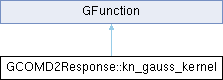GammaLib  1.7.0.dev
GCOMD2Response::kn_gauss_kernel Class Reference
Inheritance diagram for GCOMD2Response::kn_gauss_kernel:## Public Member Functions

kn_gauss_kernel (const double &ereco, const double &e0, const double &ec, const double &sigma)

double eval (const double &e)
Computes modified Klein-Nishina cross section multiplied with Gaussian. More...Public Member Functions inherited from GFunction
GFunction (void)
Void constructor. More...

GFunction (const GFunction &function)
Copy constructor. More...

virtual ~GFunction (void)
Destructor. More...

GFunctionoperator= (const GFunction &function)
Assignment operator. More...

## Protected Attributes

double m_ereco

double m_e0
Incident energy (MeV) More...

double m_ec
Compton edge energy (MeV) More...

double m_wgt
Inverse of Gaussian standard deviation (1/MeV) More...Protected Member Functions inherited from GFunction
void init_members (void)
Initialise class members. More...

void copy_members (const GFunction &function)
Copy class members. More...

void free_members (void)
Delete class members. More...

## Detailed Description

Definition at line 94 of file GCOMD2Response.hpp.

## Constructor & Destructor Documentation

 GCOMD2Response::kn_gauss_kernel::kn_gauss_kernel ( const double & ereco, const double & e0, const double & ec, const double & sigma )
inline

Definition at line 96 of file GCOMD2Response.hpp.

## Member Function Documentation

 double GCOMD2Response::kn_gauss_kernel::eval ( const double & e )
virtual

Computes modified Klein-Nishina cross section multiplied with Gaussian.

Parameters
 [in] e Energy (MeV).
Returns
Modified Klein-Nishina cross section multiplied with Gaussian

Computes the modified Klein-Nishina cross section multiplied with a Gaussian using

$KN_{\rm mod}(E|E',E_0) = \sigma_{\rm KN}(E|E_0) \times (1-C(E|E_0)) \times \exp \left( -\frac{1}{2} \frac{(E-E')^2}{\sigma^2(E')} \right)$

where

$\sigma_{\rm KN}(E|E_0) = \theta(E_c-E) \left[ \left( \frac{E/E_0}{1-E/E_0} \frac{m_e c^2}{E_0} \right)^2 - \frac{E}{E_0} + \frac{1}{1-E/E_0} \right]$

is the Klein-Nishina cross section, and

$C(E|E_0) = \theta(E-12.14) \times (1 - e^{-\mu(E|E_0) \, l(E_0)})$

is a modification, where $$\theta(x)$$ is a step function that is 1 for positive $$x$$ and 0 for non-positive $$x$$,

$\mu(E|E_0) = 0.72 \, e^{-1.28 (E_0 - E)^{0.35}} + 0.01 \, (E_0 - E) + 0.014 \, (E_0 - E)^{-2.5}$

is the total linear attenuation coefficient in NaI for all processes and

$l(E_0) = 2.9 \log( E_0 - 11.14)$

is the pathlength in the D2 module (energies are in MeV). $$E_0$$ is the position of the photo peak,

$E_c = \frac{E_0}{1 + \frac{m_e c^2}{2 E_0}}$

is the Compton edge, $$E'$$ is the reconstructed energy, $$\sigma(E')$$ is the standard deviation at the reconstructed energy, and $$E$$ is the energy over which the convolution is performed.

Implements GFunction.

Definition at line 919 of file GCOMD2Response.cpp.

References exp(), log(), m_e0, m_ec, m_ereco, m_wgt, gammalib::mec2, and pow().

## Member Data Documentation

 double GCOMD2Response::kn_gauss_kernel::m_e0
protected

Incident energy (MeV)

Definition at line 107 of file GCOMD2Response.hpp.

Referenced by eval().

 double GCOMD2Response::kn_gauss_kernel::m_ec
protected

Compton edge energy (MeV)

Definition at line 108 of file GCOMD2Response.hpp.

Referenced by eval().

 double GCOMD2Response::kn_gauss_kernel::m_ereco
protected

Definition at line 106 of file GCOMD2Response.hpp.

Referenced by eval().

 double GCOMD2Response::kn_gauss_kernel::m_wgt
protected

Inverse of Gaussian standard deviation (1/MeV)

Definition at line 109 of file GCOMD2Response.hpp.

Referenced by eval().

The documentation for this class was generated from the following files: